S k i l l
i n
A R I T H M E T I C

Lesson 10

# THE MEANING OF DIVISION

In this Lesson, we will address the following:

1. What is the problem in division?
2. How do we divide a number into equal parts?
3. What is a rate?
4. Exact versus inexact division.

Section 2

5. Some properties of division.
6. The divisor a decimal.
7. How do we divide by decomposing the dividend?

IN MULTIPLICATION we are given two numbers, and we have to find their product.

4 × 15 = ?

4 × 15 = 60.

But when we know the product, and we ask,

"What number times 15 equals 60?"

? × 15 = 60

that is called the inverse of multiplication. To answer, we have to "divide."

? = 60 ÷ 15

We have to divide 60 into groups of 15: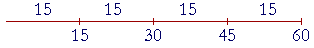We can divide 60 into four 15's.

60 ÷ 15 = 4.

"60 divided by 15 equals 4."

That means

"4 × 15 = 60."

Equivalently, we could subtract 15 from 60 four times. Multiplication is repeated addition (Lesson 8). Therefore we can think of division as repeated subtraction.

60 ÷ 15 = 4.

15 is called the divisor -- it is how 60 will be divided.  60 is called the dividend; it is the number being divided.  4 is called the quotient.

 1. What is the problem in division? 60 ÷ 15 = ?   or  ? × 15 = 60 Given two numbers, called the divisor and the dividend, we are to find the number of times the divisor is contained in the dividend. Equivalently, we are to find what number times the divisor will equal the dividend. We can also think of it as finding the number of times we could subtract the divisor from the dividend.That number of times is called the quotient.

Example 1.   What number times 6 equals 18?

Obviously, 3 × 6 = 18.  On writing this with the division sign ÷ ,

18 ÷ 6 = 3.

"18 divided by 6 equals 3."

6 -- the number to the right of the division sign ÷ -- is the divisor.  18 is the dividend.  3 is the quotient.

Here is the picture of 18 ÷ 6 :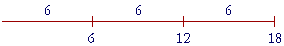6 "goes into" 18 three times.  That is,

3 × 6 = 18.

Equivalently, we could subtract 6 from 18 three times.

Here, on the other hand, is the picture of 18 ÷ 3: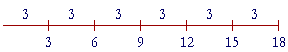18 can be divided into six 3's.

18 ÷ 3 = 6.

That is,

6 × 3 = 18.

 Dividend ÷ Divisor = Quotient Quotient × Divisor = Dividend

Note: A divisor may not be 0 -- 6 ÷ 0 -- because any number times 0 will still be 0. Therefore division by 0 is an excluded operation.

As for 0 ÷ 0, that could be any number, because any number times 0 equals 0!

Example 2.   What number times 10 will equal 72?

Answer.   Any question that asks "What number times. . .?" is a division problem.  We have to divide 72 by 10.  On separating one decimal place:

72 ÷ 10 = 7.2

(Lesson 3.)  That is,

7.2 × 10 = 72

Example 3.   How many pieces of cloth 3 yards long could you cut from a piece that is 15 yards long?

Answer.   You could cut 5 pieces -- because 5 × 3 yd = 15 yd.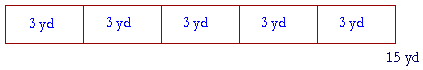15 yd ÷ 3 yd = 5.

15 yards have been divided -- broken up -- into five lengths of 3 yards each.  3 goes into 15 five times.  We could subtract 3 from 15 five times.

This problem illustrates the following point:  The dividend and divisor must be units of the same kind.  We can only divide -- repeatedly subtract -- yards from yards, dollars from dollars, hours from hours.  We cannot divide 8 apples by 2 oranges --

8 apples ÷ 2 oranges = ?

-- because no number times 2 oranges will equal 8 apples!

Example 4.   A bus is scheduled to arrive every 12 minutes.  In the course of 2 hours, how many buses will arrive?

Solution.   How many times is 12 minutes contained in 2 hours?  But the units must be the same.  Since 1 hour = 60 minutes, then 2 hours = 2 × 60 = 120 minutes.

Therefore,

120 minutes ÷ 12 minutes = 10

In the course of 2 hours, 10 buses will arrive.

(See Problem 6 at the end of the Lesson.)

Division into equal parts

 2. How do we divide a number into equal parts? Divide by the number of parts.

To divide a number into 2 equal parts,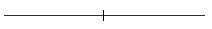divide by 2.  To divide into 3 equal parts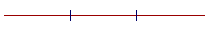divide by 3; and so on.

That is why to divide 100% into 100 equal parts -- that is, to find 1% of a number -- we divide by 100.  (Lesson 3, Question 8.)

Example 5.   If 28 people are divided into four equal parts, how many will be in each part?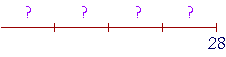Solution.   Divide by 4.   28 ÷ 4 = 7.

There will be 7 people in each part.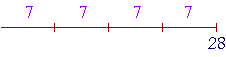But that is the picture of 28 ÷ 7!  Why does 28 ÷ 4 give the right answer?

Because of the order property of multiplication.  28 ÷ 4 = 7  means

7 × 4 = 28.

But this implies

4 × 7 = 28.

This means 28 is made up of four 7's.

Example 6.    If \$18 are divided equally among 3 people, how much will each one get?

Solution.   18 ÷ 3 = 6.  Each person will get \$6.

It seems that we are dividing units of different kinds: 18 dollars by 3 people. But if we take \$3 from \$18,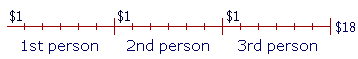and distribute \$1 to each of the 3 people; and if we do that six times -- then we have subtracted \$3 from \$18 six times!  This is our 18 ÷ 3. These are units of the same kind.

In other words,

\$18 ÷ \$3 = 6

means

6 × \$3 = \$18.

But according to the order property of multiplication,

3 × \$6 = \$18.

This means that if we divide \$18 into three equal parts, each part is \$6.

Any problem in which we appear to be dividing units of different kinds is called a rate problem, as we are about to see.

Rates

 3. What is a rate? A rate is a relationship between units of different kinds. Dollars per person. Miles per hour. And so on.

A rate is typically indicated by per, which means for each or in each.

Per always indicates division.

Example 7.   In a certain country, the unit of currency is the corona. With \$11 Ana was able to buy 55 coronas.  What was the rate of exchange?  That is, how many coronas per dollar?

Solution.   Follow the sequence:  coronas per dollar:  55 ÷ 11 = 5.

The rate of exchange was 5 coronas per dollar.

Any rate problem -- dollars per person, miles per hour -- is equivalent to dividing a number into equal parts. Rate problems can therefore can be analyzed in the same manner as the Example above. To preserve the meaning of division, we must divide units of the same kind, even though that is not how it appears.

Exact versus inexact division

 4. Which numbers will be exactly divisible by a given number? The multiples of that number.

The numbers exactly divisible by 3 are the multiples of 3:

3,  6,  9,  12, and so on.

And since these are divisible by 3, so are

30,  60,  90,  120, . . .

300,  600,  900,  1200, . . .

The numbers exactly divisible by 8 are the multiples of 8:

8,  16,  24,  32, . . .

80,  160,  240,  320, . . .

800,  1600,  2400,  3200, . . .

The figure below illustrates that 8 goes into 32 exactly.  32 is a multiple of 8.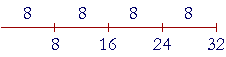But 8 does not go into 35 exactly:35 is not a multiple of 8.  There is a remainder of 3.

35 ÷ 8 = 4 R 3

The remainder is what we have to add to the multiple of 8 to get 35.

35 = 4 × 8  +  3

Possible remainders

Say that there is a large group of people, and we want to divide them into groups of 5.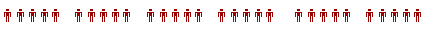But say we discover that there is not an exact multiple of 5.  Then how many people might we not be able to group?  How many people might remain?

Answer:  Either 1, or 2, or 3, or 4.  Because if more than 4 remained, we could make another group of 5!

The point is:

The remainder is always less than the divisor.

If we divide by 5, then the possible remainders are 1, 2, 3, or 4.

Example 8.

a)   If 7 is the divisor, what are the possible remainders?

Answer.   1, 2, 3, 4, 5, 6.

b)   How many 7's are there in 61?

Answer.   8.  And there is a remainder of 5.

61 ÷ 7 = 8 R 5

That is,

61 = 8 × 7  +  5

Example 9.   Prove:   47 ÷ 9 = 5 R 2

Proof.   47 = 5 × 9  +  2.

Example 10.   Divide 53 by 8.  Write the whole number quotient and the remainder.

Answer.   53 ÷ 8 = 6 R 5

8 goes into 53 six (6) times:  48.  What must we add to 48 to get 53?

5.

48 + 5 = 53.

5 is the remainder.

The division bar

In what follows, we will signify division in this way:

 16 8 =  2

"16 divided by 8 is 2."

 Dividend  Divisor =  Quotient

The test is:

Quotient × Divisor = Dividend

The short horizontal line is called the division bar. The division bar is also used to signify a fraction, because a fraction indicates division of the numerator by the denominator. (Lessons 19 and 23.)  We also use the division bar to signify the ratio of two numbers. (Lesson 16.)

 Example 11. 280  7 = ?

"280 divided by 7 is what number?"

Answer.  Ignore the 0.  7 goes into 28 four (4) times.  Therefore 7 goes into 280 forty (40) times.

 280  7 = 40.

40 times 7 is 280.

In other words, since 28 is divisible by 7, then so is '28' followed by any number of 0's.

280   2800   28,000   280,000  . . .

 Example 12. 5400   9 = ?

Answer.  600.  Because 600 × 9 = 5400.

 Example 13. 246100 = 2.46

This is 246 ÷ 100.  (Lesson 3)

 Example 14.    5 ÷ 8  = 58
 5 ÷ 8 is simply the fraction 58 -- which we can interpret as 5 ÷ 8

In the next Lesson we will see how to express 5 ÷ 8 as a decimal.

In Lesson 31, Prime numbers, we will learn about the divisors of a number.

Section 2:  Some properties of division

www.proyectosalonhogar.com